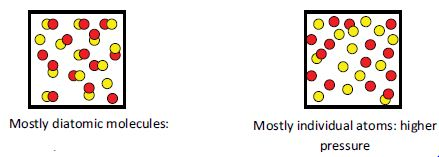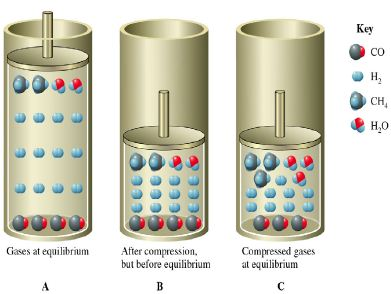# Effects of Pressure Change in terms of Le Chatelier’s Principle

Le Chatelier’s Principle can also be used to predict how changing the pressure can also alter the position of equilibrium. The pressure of a gas is related to the number of moles in a certain volume. So we can make the pressure change by reducing or increasing the volume of the reaction vessel.

If the total number of molecules of gaseous product is different to the total number of molecules of gaseous reactant, then the pressure will change by itself if the equilibrium position shifts.

If the products in a gaseous reaction contain fewer moles of gas than the reactants, the products would require less space. So, reducing the volume of the reaction vessel would, therefore, favour the products.Consider the following reaction:

CO (g) + 3H2 (g) ↔ CH4 (g) + H2O (g)

The figure below shows the above reaction at equilibrium and its compressionConversely, if the reactants require less volume (i.e. fewer moles of gaseous reactant) then decreasing the volume of the reaction vessel would shift the equilibrium to the left (i.e. toward the reactants). Literally squeezing the reaction will cause a shift in the equilibrium toward the fewer moles of gas. Reducing the pressure in the reaction vessel by increasing its volume would have the opposite effect.

Le Chatelier’s principle can be applied to pressure changes in the following way: “when a system in a chemical equilibrium is disturbed by a change of pressure, the equilibrium will shift in a way that tends to counteract this change:’ So, if we increase the pressure, the equilibrium will shift in whichever direction will reduce the pressure and vice versa.

A pressure change caused by changing the volume of the reaction vessel will affect the yield of products in a gaseous reaction only if there is a change in the total moles of gas present. If the number of moles of gaseous product equals the number of moles of gaseous reactant, changing the volume of the reaction vessel will have no effect on the position of the equilibrium.